﻿ 基于变值图示判定RNN基因序列分类器的稳定性

# 基于变值图示判定RNN基因序列分类器的稳定性Stability of RNN Classified Gene Sequence on Variant Maps

Abstract: The recurrent neural network (RNN) has an excellent role to analyze the data characteristics of integer sequences. Using the principle of feature classification, the selected gene sequences are pre-classified, and subsequent processes are performed on the classified sequences. In this paper, the pre-classified gene sequences are segmented and shifted. For the sequence operation after classification, the gene sequence data sets with different shift-length values are obtained, and the different data sets are respectively used as the inputs of the RNN classifier. The sequences of different detection data sets are replaced to obtain the final visualized sequence data set. The variant maps are provided to show a series of visualization results of the variant probability statistics. The stability of the RNN classifier is analyzed through comparison and analysis of the variant maps and other diagrams. Multiple substitution relationships in the replacement operation change lengths of the shift operations to provide a variety of visualization and comprehensive cross-comparisons to support the analysis and in-depth exploration of the stability problems of the RNN classifier.

1. 引言

2. 体系结构

3. 核心处理模块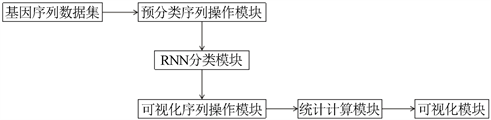Figure 1. Flow Chart of Data processing

3.1. 预分类序列操作模块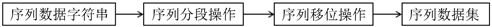Figure 2. Operation diagram of pre-classification sequence

3.2. RNN分类模块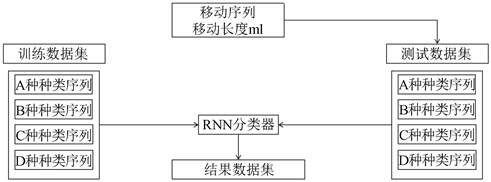Figure 3. Flow Chart of Classifier classification

3.3. 可视化序列操作模块

1) RY：嘌呤(R) = A、G；嘧啶(Y) = C、T；

2) MK：氨基(M) = A、C；羧基(K) = G、T；

3) SW：强氢键(S) = G、C；弱氢键(W) = A、T；

1) RY：‘RR’，‘RY’，‘YR’，‘YY’；

2) MK：‘KK’，‘KM’，‘MK’，‘MM’；

3) SW：‘SS’，‘SW’，‘WS’，‘WW’；

3.4. 统计计算模块

3.5. 可视化模块

3.5.1. 序列差异

$ldis{t}_{a,b}\left(i,j\right)=\left\{\begin{array}{l}\mathrm{max}\left(i,j\right),\mathrm{min}\left(i,j\right)=0\\ \mathrm{min}\left\{\begin{array}{l}ldis{t}_{a,b}\left(i-1,j\right)+1\\ ldis{t}_{a,b}\left(i,j-1\right)+1\\ ldis{t}_{a,b}\left(i-1,j-1\right)+1\end{array}\right\},otherwise\end{array}\right\}$

$r=\frac{\left(sum-ldis{t}_{a,b}\right)}{sum}$

$sum=m+n$

3.5.2. 分类结果统计

3.5.3. 分类结果数据集统计

4. 数据简介

5. 结果展示与分析

5.1. 结果展示

5.1.1. 分类结果数据集统计

5.1.2. 分类结果统计可视化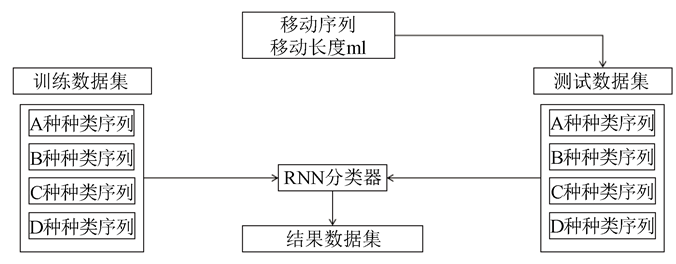Figure 4. Flow Chart of Classifier classification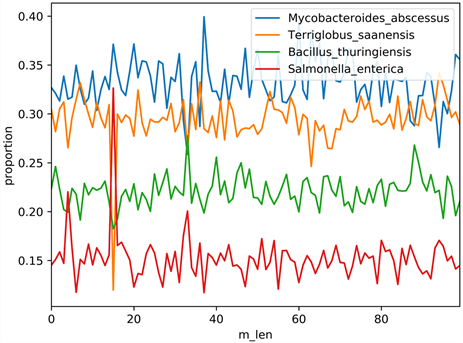Figure 5. Proportion diagram

5.1.3. 分类结果数据集可视化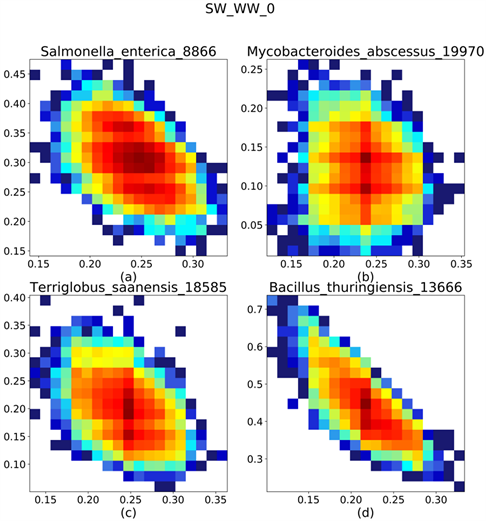Figure 6. Result of 0 Length-Shift

1) 移动长度(mlen)为1可得如图“图7”；

2) 移动长度(mlen)为21可得如图“图8”；

3) 移动长度(mlen)为41可得如图“图9”；

4) 移动长度(mlen)为61可得如图“图10”；

5) 移动长度(mlen)为81可得如图“图11”；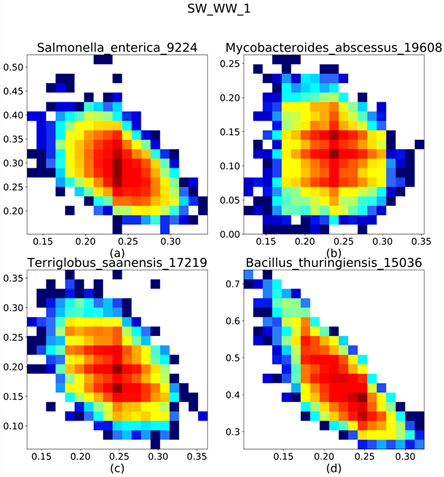Figure 7. Result of 1 Length-Shift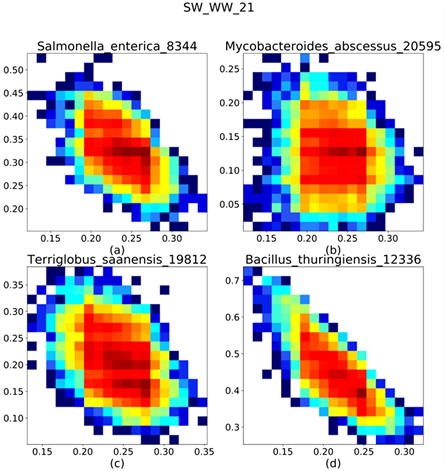Figure 8. Result of 21 Length-Shift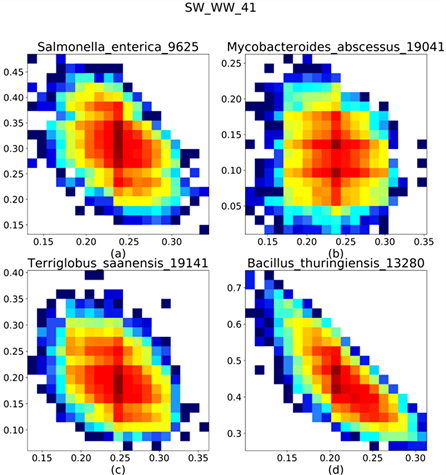Figure 9. Result of 41 Length-Shift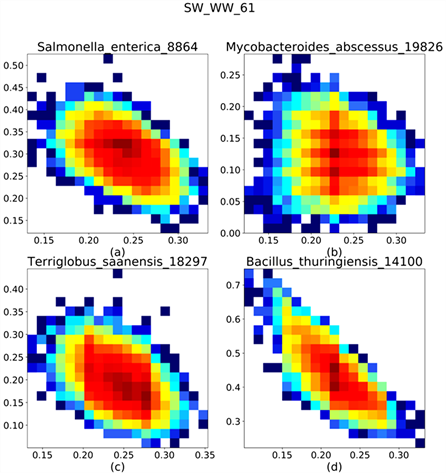Figure 10. Result of 61 Length-Shift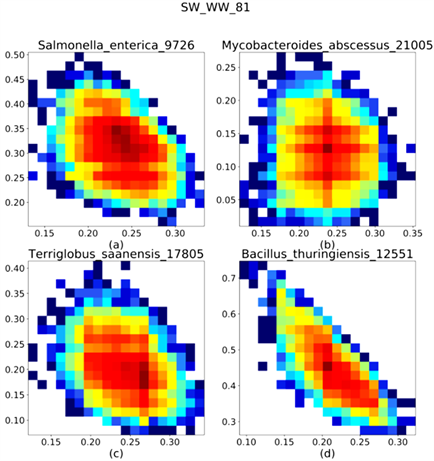Figure 11. Result of 81 Length-ShiftTable 1. System resulting data of standard experiment

5.2. 结果分析

RNN分类器在序列元素位置变化的情况下，分类操作时仍然是稳定的，序列数据中元素的位置改变在一定范围内对RNN分类器的影响是十分有限的。

6. 总结

NOTES

*通讯作者。

 Salehinejad, H., Sankar, S., Barfett, J., Colak, E. and Valaee, S. (2018) Recent Advances in Recurrent Neural Networks.
https://arxiv.org/pdf/1801.01078.pdf

 杨丽, 吴雨茜, 王俊丽, 刘义理. 循环神经网络研究综述[J]. 计算机应用, 2018, 38(S2): 1-6

 Aungiers, J. (2018) Tim Series Prediction Using LSTM Deep Neural Networks.
https://www.altumintelligence.com/articles/a/Time-Series-Prediction-Using-LSTM-Deep-Neural-Networks

 黄有为, 高燕. 基于循环神经网络的金融数据预测系统[J]. 软件导刊, 2019, 18(1): 28-33, 226.

 韩丰, 龙明盛, 李月安, 等. 循环神经网络在雷达临近预报中的应用[J]. 应用气象学报, 2019, 30(1): 61-69.

 唐美丽, 胡琼, 马廷淮. 基于循环神经网络的语音识别研究[J]. 现代电子技术, 2019, 42(14): 152-156.

 郑智捷, 郑昊航. 变值测量结构及其可视化统计分布[J]. 光子学报, 2011, 40(9): 1397-1404.

 Zheng, J. (2011) Conditional Probability Statistical Distributions in Variant Measurement Simulations. Acta Photonica Sinica, 40, 1662-1666.
https://doi.org/10.3788/gzxb20114011.1662

 Zheng, J. (2019) Novel Pseudorandom Number Generation Using Variant Logic Framework. Variant Construction from Theoretical Foundation to Applications, Springer, Singapore, 289-295.
https://doi.org/10.1007/978-981-13-2282-2_18 .

 Zheng, J. (2019) Variant Logic Construction under Permutation and Complementary Operations on Binary Logic. In: Zheng, J., Ed., Variant Construction from Theoretical Foundation to Applications, Springer, Singapore, 3-21.
https://doi.org/10.1007/978-981-13-2282-2_1

 Levenshtein, V. (1966) Binary Codes Capable of Correcting Deletions, Insertions, and Reversals. Soviet Physics Doklady, 10, 707-710.

 赵作鹏, 尹志民, 王潜平, 等. 一种改进的编辑距离算法及其在数据处理中的应用[J]. 计算机应用, 2009, 29(2): 424-426.

Top